13.3 Insertion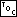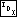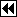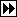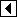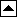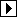The only difference between this code and <BST item insertion function 32> is that we set n's parent pointer after insertion.

```490. <PBST item insertion function 490> =
void **pbst_probe (struct pbst_table *tree, void *item) {
struct pbst_node *p, *q; /* Current node in search and its parent. */
int dir;                 /* Side of q on which p is located. */
struct pbst_node *n;     /* Newly inserted node. */

assert (tree != NULL && item != NULL);

<Step 1: Search PBST tree for insertion point 491>
<Step 2: Insert PBST node 492>

return &n->pbst_data;
}
```

This code is included in 489.

```491. <Step 1: Search PBST tree for insertion point 491> =
for (q = NULL, p = tree->pbst_root; p != NULL; q = p, p = p->pbst_link[dir])   {
int cmp = tree->pbst_compare (item, p->pbst_data, tree->pbst_param);
if (cmp == 0)
return &p->pbst_data;
dir = cmp > 0;
}
```

This code is included in 490 and 555.

```492. <Step 2: Insert PBST node 492> =
n = tree->pbst_alloc->libavl_malloc (tree->pbst_alloc, sizeof *p);
if (n == NULL)
return NULL;

tree->pbst_count++;